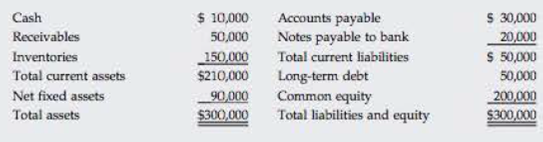Chapter 4, Problem 15PFundamentals of Financial Manageme...

15th Edition
Eugene F. Brigham + 1 other
ISBN: 9781337395250

Solutions

Chapter
SectionFundamentals of Financial Manageme...

15th Edition
Eugene F. Brigham + 1 other
ISBN: 9781337395250
Textbook Problem

RETURN ON EQUITY AND QUICK RATIO Lloyd Inc. has sales of $200,000, a net income of$15,000, and the following balance sheet:The new owner thinks that inventories are excessive and can be lowered to the point where the current ratio is equal to the industry average, 25×, without affecting sales or net income. If inventories are sold and not replaced (thus reducing the current ratio to 25×); if the funds generated are used to reduce common equity (stock can be repurchased at book value); and if no other changes occur, by how much will the ROE change? What will be the firm’s new quick ratio?

Summary Introduction

To identify: The change in return on equity and new quick ratio.

Quick Ratio: A part of liquidity ratios, quick ratio reflects the ability to oblige the short term debts of a company. It is calculated based on the liquid assets and current liabilities; a company has in an accounting period.

Return on Equity: Return on equity represents the amount earned as return by equity share holders; it can be calculated by dividing earnings available for equity share holders to total equity capital.

Explanation

Computation of return on equity

Items required for the calculation of return on equity are net income and common equity.

Given,

Net income is $15,000. Common equity is$200,000.

Formula to calculate return on equity ratio,

ROE=NetincomeCommonequity

Where,

• ROE is return on equity.

Substitute $15,000 for net income and$200,000 for common equity in the above formula,

ROE=$15,000$200,000=0.075

Hence, the return on equity is 0.075 or 7.5%.

Compute the quick ratio

Given,

The current assets are $210,000. The inventories are$150,000.

The current liabilities are $50,000. Formula to compute quick ratio, Quickratio=CurrentassetsInventoriesCurrentliabilities Substitute$210,000 for current assets, $150,000 for inventories and$50,000 for current liabilities in the above formula,

Quickratio=$210,000$150,000$50,000=$60,000$50,000=1.2times The quick ratio is 1.2 times. In order to compute new quick ratio, old current ratio, new current assets and new return on equity need to calculate. Computation of old current ratio The items required for the calculation of current ratio are current liabilities and current assets. Given, Current assets are$210,000.

Current liabilities are $50,000. Formula to calculate current ratio, Currentratio=CurrentassetsCurrentliabilities Substitute$210,000 for current assets and $50,000 for current liabilities in the above formula, Currentratio=$210,000$50,000=4.2times Hence, old current ratio is 4.2 times. The new current ratio which is required to take is 2.5 times. Compute the change in assets due to the current ratio as 2.5 times. The current liabilities are$50,000. (Given)

The current ratio is 2.5 times.

Formula to calculate new current assets derives from the formula of current ratio,

Currentratio=CurrentassetsCurrentliabilitiesCurrentassets=Currentratio×Currentliabilities

Substitute $50,000 for current liabilities and 2.5 for current ratio in the above formula, Currentassets=2.5×$50,000=$125,000 The new current assets are$125,000.

The difference between the currents assets refers the value of sold inventory.

Compute the sold inventory due to change in current assets

The current assets are $210,000. (Given) The new current assets are$125,000. (Calculated)

Formula to calculate the sold inventory,

Soldinventory=OldcurrentassetsNewcurrentassets

Substitute $210,000 for old current assets and$125,000 for new current assets in the above formula,

Soldinventory=$210,000$125,000=$85,000 The value of inventor is curtailed by the$85,000

Still sussing out bartleby?

Check out a sample textbook solution.

See a sample solution

The Solution to Your Study Problems

Bartleby provides explanations to thousands of textbook problems written by our experts, many with advanced degrees!

Get Started

Find more solutions based on key concepts